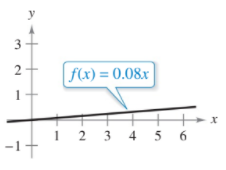# ?Precalculus or Calculus In Exercises 1-5, decide whether the problem can be solved using precalculus or whether calculus is required. If the problem c

Chapter 2, Problem 4

#### (choose chapter or problem)

Precalculus or Calculus In Exercises 1-5, decide whether the problem can be solved using precalculus or whether calculus is required. If the problem can be solved using precalculus, solve it. If the problem seems to require calculus, explain your reasoning and use a graphical or numerical approach to estimate the solution.

A bicyclist is riding on a path modeled by the function f(x) = 0.08x, where x and f(x) are measured in miles (see figure). Find the rate of change of elevation at x = 2.Unfortunately, we don't have that question answered yet. But you can get it answered in just 5 hours by Logging in or Becoming a subscriber.